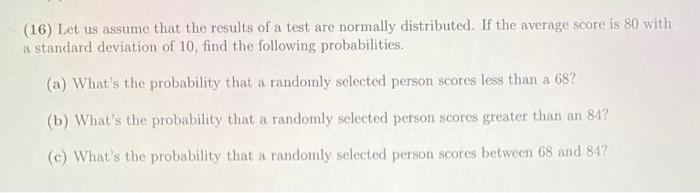Home / Expert Answers / Statistics and Probability / 16-let-us-assume-that-the-results-of-a-test-are-normally-distributed-if-the-average-score-is-80-pa877

# (Solved): (16) Let us assume that the results of a test are normally distributed. If the average score is 80 ...(16) Let us assume that the results of a test are normally distributed. If the average score is 80 with a standard deviation of 10 , find the following probabilities. (a) What's the probability that a randomly selected person scores less than a 68 ? (b) What's the probability that a randomly selected person scores greater than an 84 ? (c) What's the probability that a randomly selected person scores between 68 and

We have an Answer from Expert